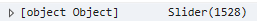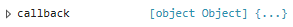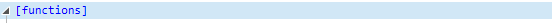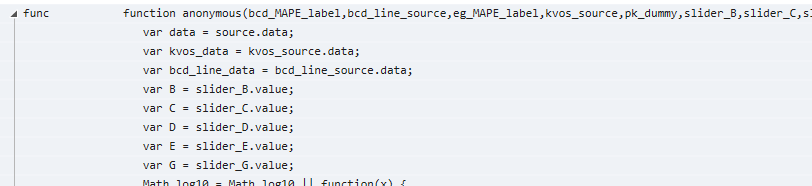# Force a callback from a different javascript callback

Lets say i have a java callback, which is called on a tap event on a figure.

In this call back i change a different source. i make part of it selected, like that:

``````source.selected['1d'].indices = 
``````
``````
``````
``````
``````
``````On that source i have a different callback:
``````
``````
``````

source.callback = some_selection_callback

My question is, how from the first callback, after changing the selected indices, can i force a call to the second callback “some_selection_callback”

the Selection was changed, but the callback isnt being called.

how from the java script code can i force him to be called?

Thanks!

You search something like this?
import numpy as np

from bokeh.plotting import Figure, show

from bokeh.models import CustomJS, ColumnDataSource

from bokeh.events import Tap

points = np.random.rand(50, 2)

cds = ColumnDataSource(data=dict(x=points[:, 0], y=points[:, 1]))

p = Figure(x_range=(0, 1), y_range=(0, 1), tools="")

p.scatter(x=‘x’, y=‘y’, source=cds)

cb_to_make_selection = CustomJS(args={‘cds’: cds}, code="""

function getRandomInt(max) {

return Math.floor(Math.random() * Math.floor(max));

}

cds.selected.indices = [getRandomInt(cds.get_length()-1)]

“”")

p.js_on_event(Tap, cb_to_make_selection)

cb_on_selection = CustomJS(code=“alert(‘triggered by change one selection’)”)

cds.selected.js_on_change(‘indices’, cb_on_selection)

show§

When you tap on the plot a point is randomly selected and then an alert pop

···

Le dimanche 20 janvier 2019 15:16:34 UTC+1, Tamir Gefen a écrit :

Lets say i have a java callback, which is called on a tap event on a figure.

In this call back i change a different source. i make part of it selected, like that:

``````source.selected['1d'].indices = 
``````
``````

``````
``````

``````
``````On that source i have a different callback:
``````
``````

``````

source.callback = some_selection_callback

My question is, how from the first callback, after changing the selected indices, can i force a call to the second callback “some_selection_callback”

the Selection was changed, but the callback isnt being called.

how from the java script code can i force him to be called?

Thanks!

not exactly. my question is how can you force the call for the cds.selected defined callback. if will be called when you tap on the plot, that i understand. but i want to be able to call it myself from a different callback javascrip

···

On Sunday, January 20, 2019 at 7:08:28 PM UTC+2, Xavier Artusi wrote:

You search something like this?
import numpy as np

from bokeh.plotting import Figure, show

from bokeh.models import CustomJS, ColumnDataSource

from bokeh.events import Tap

points = np.random.rand(50, 2)

cds = ColumnDataSource(data=dict(x=points[:, 0], y=points[:, 1]))

p = Figure(x_range=(0, 1), y_range=(0, 1), tools="")

p.scatter(x=‘x’, y=‘y’, source=cds)

cb_to_make_selection = CustomJS(args={‘cds’: cds}, code="""

function getRandomInt(max) {

return Math.floor(Math.random() * Math.floor(max));

}

cds.selected.indices = [getRandomInt(cds.get_length()-1)]

“”")

p.js_on_event(Tap, cb_to_make_selection)

cb_on_selection = CustomJS(code=“alert(‘triggered by change one selection’)”)

cds.selected.js_on_change(‘indices’, cb_on_selection)

show§

When you tap on the plot a point is randomly selected and then an alert pop

Le dimanche 20 janvier 2019 15:16:34 UTC+1, Tamir Gefen a écrit :

Lets say i have a java callback, which is called on a tap event on a figure.

In this call back i change a different source. i make part of it selected, like that:

``````source.selected['1d'].indices = 
``````
``````

``````
``````

``````
``````On that source i have a different callback:
``````
``````

``````

source.callback = some_selection_callback

My question is, how from the first callback, after changing the selected indices, can i force a call to the second callback “some_selection_callback”

the Selection was changed, but the callback isnt being called.

how from the java script code can i force him to be called?

Thanks!

Tamir,

this is how a accomplished it.

First, I have a CustomJS callback (code omitted since it has no use here):

``````sliders_callback = CustomJS(args = dict(source = gen_plot_lines_cds,
``````

kvos_source = kvos_plot_cds,

bcd_line_source = bcd_line_cds,

slider_B = slider_B,

slider_C = slider_C,

slider_D = slider_D,

slider_E = slider_E,

slider_G = slider_G,

pk_dummy = pk_dummy,

bcd_MAPE_label = bcd_MAPE_label,

``````                                         eg_MAPE_label = eg_MAPE_label), code="""...""")
``````

Then I assign this one to slider callback:

slider_C.callback = sliders_callback

Then I can call this callback func in another CustomJS instance assigned to another model:

``````cancel_changes_btn.callback = CustomJS(args=dict(slider_B = slider_B,

slider_C = slider_C,

slider_D = slider_D,

slider_E = slider_E,

slider_G = slider_G,

B=B,

C=C,

D=D,

E=E,

G=G,

source = gen_plot_lines_cds,

kvos_source = kvos_plot_cds,
``````

pk_dummy = pk_dummy,

``````                                        bcd_line_source = bcd_line_cds,

bcd_MAPE_label = bcd_MAPE_label,
``````

eg_MAPE_label = eg_MAPE_label),code="""

slider_B.value = B;

``````    slider_C.value = C;

slider_D.value = D;

slider_E.value = E;

slider_G.value = G;

slider_C.callback.func(bcd_MAPE_label, bcd_line_source, eg_MAPE_label, kvos_source, pk_dummy,

slider_B,slider_C,slider_D,slider_E,slider_G, source, null,null,null,null);

""")
``````

It looks scary but I don’t have another example at the moment.

···

понедельник, 21 января 2019 г., 12:50:09 UTC+3 пользователь Tamir Gefen написал:

not exactly. my question is how can you force the call for the cds.selected defined callback. if will be called when you tap on the plot, that i understand. but i want to be able to call it myself from a different callback javascrip

On Sunday, January 20, 2019 at 7:08:28 PM UTC+2, Xavier Artusi wrote:

You search something like this?
import numpy as np

from bokeh.plotting import Figure, show

from bokeh.models import CustomJS, ColumnDataSource

from bokeh.events import Tap

points = np.random.rand(50, 2)

cds = ColumnDataSource(data=dict(x=points[:, 0], y=points[:, 1]))

p = Figure(x_range=(0, 1), y_range=(0, 1), tools="")

p.scatter(x=‘x’, y=‘y’, source=cds)

cb_to_make_selection = CustomJS(args={‘cds’: cds}, code="""

function getRandomInt(max) {

return Math.floor(Math.random() * Math.floor(max));

}

cds.selected.indices = [getRandomInt(cds.get_length()-1)]

“”")

p.js_on_event(Tap, cb_to_make_selection)

cb_on_selection = CustomJS(code=“alert(‘triggered by change one selection’)”)

cds.selected.js_on_change(‘indices’, cb_on_selection)

show§

When you tap on the plot a point is randomly selected and then an alert pop

Le dimanche 20 janvier 2019 15:16:34 UTC+1, Tamir Gefen a écrit :

Lets say i have a java callback, which is called on a tap event on a figure.

In this call back i change a different source. i make part of it selected, like that:

``````source.selected['1d'].indices = 
``````
``````

``````
``````

``````
``````On that source i have a different callback:
``````
``````

``````

source.callback = some_selection_callback

My question is, how from the first callback, after changing the selected indices, can i force a call to the second callback “some_selection_callback”

the Selection was changed, but the callback isnt being called.

how from the java script code can i force him to be called?

Thanks!

correct me if im wrong, the line i was actually looking for is:

“slider_C.callback.func()”?

this what calls the slider_c callback from the JS code?

···

On Sunday, January 20, 2019 at 4:16:34 PM UTC+2, Tamir Gefen wrote:

Lets say i have a java callback, which is called on a tap event on a figure.

In this call back i change a different source. i make part of it selected, like that:

``````source.selected['1d'].indices = 
``````
``````

``````
``````

``````
``````On that source i have a different callback:
``````
``````

``````

source.callback = some_selection_callback

My question is, how from the first callback, after changing the selected indices, can i force a call to the second callback “some_selection_callback”

the Selection was changed, but the callback isnt being called.

how from the java script code can i force him to be called?

Thanks!

Exactly! Here’s the JS structure of my “slider_C”:That is exactly the code assigned with CustomJS. Just make sure you pass all the args within your function:

function anonymous(bcd_MAPE_label,bcd_line_source,eg_MAPE_label,kvos_source,pk_dummy,slider_B,slider_C,slider_D,slider_E,slider_G,source, cb_obj,cb_data,require,exports)

Those marked red are part of callback func as well, they are defined by bokeh implicitly.

···

понедельник, 21 января 2019 г., 16:23:27 UTC+3 пользователь Tamir Gefen написал:

correct me if im wrong, the line i was actually looking for is:

“slider_C.callback.func()”?

this what calls the slider_c callback from the JS code?

On Sunday, January 20, 2019 at 4:16:34 PM UTC+2, Tamir Gefen wrote:

Lets say i have a java callback, which is called on a tap event on a figure.

In this call back i change a different source. i make part of it selected, like that:

``````source.selected['1d'].indices = 
``````
``````

``````
``````

``````
``````On that source i have a different callback:
``````
``````

``````

source.callback = some_selection_callback

My question is, how from the first callback, after changing the selected indices, can i force a call to the second callback “some_selection_callback”

the Selection was changed, but the callback isnt being called.

how from the java script code can i force him to be called?

Thanks!